you are here:/ News & Insights / Engineering Advantage Blog / How Do You Find the Peak Stress in a Harmonic Response Analysis?

# How Do You Find the Peak Stress in a Harmonic Response Analysis?October 30, 2015 By: Eric Stamper

This question has come up a couple times recently, so I'll describe a general approach that can be used in this post.

The visual example to go along with this explanation is a centrifuge frame that has three legs, shown in Figure 1, above. Imagine that the spinning rotor (not shown) has an imbalance that causes stress in the frame over a speed range. What we are looking to find is the peak value of stress in the frame, the speed at which this happens, and the distribution of stress when this peak value occurs. A harmonic response analysis with a rotating imbalance force can be used to perform this type of simulation.

The loading in this case can be described mathematically as: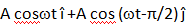, where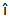and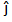are unit vectors in 2 mutually orthogonal directions, X and Y, A is the load amplitude (which varies as the square of the rotational speed), and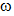is the rotational speed in rad./sec. In this case of a rotating imbalance, the phase angle between the loading in the X and Y directions is 90 degrees. That is, the Y-direction sees the peak value of the load 90 degrees out of phase with the X-direction, see Figure 2. The combination of these two orthogonal forces produces a uniform amplitude of radial load at any circumferential position.

Figure 2. Force and Phase Relationships

## harmonic2.pngAs the rotor spins up to its maximum speed, the frequency and amplitude of the rotating force increase. For a linear system, the steady state response to this loading is also sinusoidal, at the same frequency as the loading. It takes the form of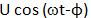, where U is the amplitude of a response quantity of interest, say stress, and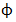is the phase angle of this response. Using our example, the phase angle corresponds to the angular position of the imbalance at the instant in time when the response quantity reaches its maximum value.

So, how do we achieve our goal of determining the speed (frequency) and phase angle (direction of the imbalance force) at which the peak stress occurs in the model?

A general approach is to look at a frequency response plot, sometimes called a Bode plot, which shows a graph of the peak amplitude of the quantity of interest (in our case stress) versus frequency along with the phase angle at which peak amplitude occurs, at a specific location in the model. Usually, the peak response will be at one of the natural frequencies of the structure. Once the critical frequency and phase angle have been determined, a contour plot of stress can be made, which represents a snapshot in time of the stress distribution in the entire model when the peak stress occurs.

Some FEA codes, like ANSYS Mechanical, simplify this process. In ANSYS, a composite Bode plot is easily created, which summarizes the peak stress over all the nodes in the model at each frequency, and the phase angle at which it occurs (Figure 3). For this example, we see from the Bode plot that the peak stress from the queried locations of 146 ksi. occurs at a frequency of 344.65 Hz and a phase angle of 1.18°.  However, we don’t know from the composite Bode plot where the peak stress is located. To determine this information, a contour plot of the stresses at this frequency and phase angle can be easily generated, which tells the whole story as shown in Figure 4. The X-axis of the triad is oriented at the phase angle of 1.184° to illustrate the imbalance force direction and the translucent frame is shown with exaggerated displacements.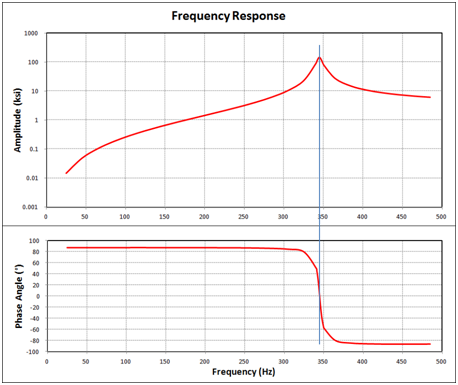This simple, two-click, process can greatly reduce the time and effort required to get to the important results from a harmonic response analysis and ensure that a critical stress is not overlooked.

Figure 4: Contour Plot of Max. Stress from Bode Plot

## harmonic4.pngI look forward to learning about other methods being used in order to capture peak stress values in harmonic response analyses. Please send along your comments.